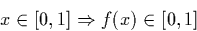Next: Dyadic Arithmetic Up: Theoretical Analysis Previous: Division

## Logistic Map

The logistic map is a quadratic map defined as f(x) = Ax(1-x) where A is a real constant. For some values of A, iterating the function produce chaotic behaviour (see  p.130-139). For the value 4, the behaviour is chaotic, and the function maps the interval [0,1] onto itself. The chaotic nature of the system produced has the effect that when computed using floating point arithmetic we rapidly get erroneous results. This is illustrated in section 2.1.1.

We examine the lookahead required to compute this using our implementation of exact real arithmetic supposing a is exactly equal to 4. Let f(x) = 4x(1-x). Observe thatso we could compute this using either the stream representation alone or the (mantissa, exponent). In fact, the lookahead can be smaller for the (mantissa, exponent) case if we implement multiplication by four by simply adding two to the exponent. This is a hollow saving, however, because in order to obtain the same precision we must evaluate more of the exponent. For example, if the exponent is n, and we require m signed binary digits of the result past the binary point, we must in fact evaluate m+n digits of the mantissa.Next: Dyadic Arithmetic Up: Theoretical Analysis Previous: Division
Martin Escardo
5/11/2000